# How to subtract in Excel: formula for numbers, percentages, dates and times

The tutorial shows how to do subtraction in Excel by using the minus sign and SUM function. You will also learn how to subtract cells, entire columns, matrices and lists.

Subtraction is one of the four basic arithmetic operations, and every primary school pupil knows that to subtract one number from another you use the minus sign. This good old method works in Excel too. What kind of things can you subtract in your worksheets? Just any things: numbers, percentages, days, months, hours, minutes and seconds. You can even subtract matrices, text strings and lists. Now, let's take a look at how you can do all this.

## Subtraction formula in Excel (minus formula)

For the sake of clarity, the SUBTRACT function in Excel does not exist. To perform a simple subtraction operation, you use the minus sign (-).

The basic Excel subtraction formula is as simple as this:

=number1-number2

For example, to subtract 10 from 100, write the below equation and get 90 as the result:

`=100-10`

To enter the formula in your worksheet, do the following:

1. In a cell where you want the result to appear, type the equality sign (=).
2. Type the first number followed by the minus sign followed by the second number.
3. Complete the formula by pressing the Enter key.

Like in math, you can perform more than one arithmetic operation within a single formula.

For example, to subtract a few numbers from 100, type all those numbers separated by a minus sign:

`=100-10-20-30`

To indicate which part of the formula should be calculated first, use parentheses. For example:

`=(100-10)/(80-20)`

The screenshot below shows a few more formulas to subtract numbers in Excel: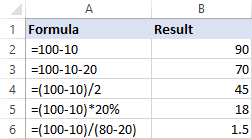## How to subtract cells in Excel

To subtract one cell from another, you also use the minus formula but supply cell references instead of actual numbers:

=cell_1 - cell_2

For example, to subtract the number in B2 from the number in A2, use this formula:

`=A2-B2`

You do not necessarily have to type cell references manually, you can quickly add them to the formula by selecting the corresponding cells. Here's how:

1. In the cell where you want to output the difference, type the equals sign (=) to begin your formula.
2. Click on the cell containing a minuend (a number from which another number is to be subtracted). Its reference will be added to the formula automatically (A2).
3. Type a minus sign (-).
4. Click on the cell containing a subtrahend (a number to be subtracted) to add its reference to the formula (B2).
5. Press the Enter key to complete your formula.

And you will have a result similar to this: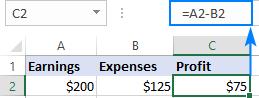## How to subtract multiple cells from one cell in Excel

To subtract multiple cells from the same cell, you can use any of the following methods.

#### Method 1. Minus sign

Simply type several cell references separated by a minus sign like we did when subtracting multiple numbers.

For example, to subtract cells B2:B6 from B1, construct a formula in this way:

`=B1-B2-B3-B4-B5-B6`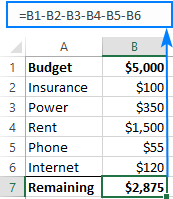#### Method 2. SUM function

To make your formula more compact, add up the subtrahends (B2:B6) using the SUM function, and then subtract the sum from the minuend (B1):

`=B1-SUM(B2:B6)`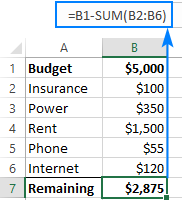#### Method 3. Sum negative numbers

As you may remember from a math course, subtracting a negative number is the same as adding it. So, make all the numbers you want to subtract negative (for this, simply type a minus sign before a number), and then use the SUM function to add up the negative numbers:

`=SUM(B1:B6)`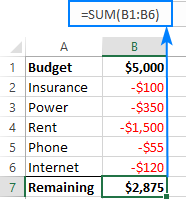## How to subtract columns in Excel

To subtract 2 columns row-by-row, write a minus formula for the topmost cell, and then drag the fill handle or double-click the plus sign to copy the formula to the entire column.

As an example, let's subtract numbers in column C from the numbers in column B, beginning with row 2:

`=B2-C2`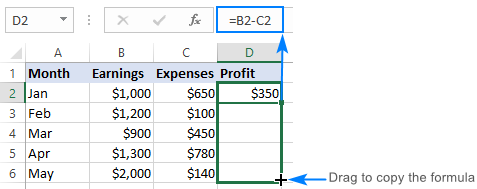Due to the use of relative cell references, the formula will adjust properly for each row: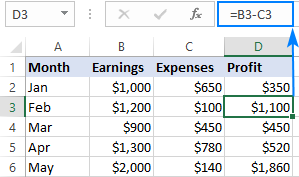## Subtract the same number from a column of numbers

To subtract one number from a range of cells, enter that number in some cell (F1 in this example), and subtract cell F1 from the first cell in the range:

`=B2-\$F\$1`

The key point is to lock the reference for the cell to be subtracted with the \$ sign. This creates an absolute cell reference that does not change no matter where the formula is copied. The first reference (B2) is not locked, so it changes for each row.

As the result, in cell C3 you will have the formula =B3-\$F\$1; in cell C4 the formula will change to =B4-\$F\$1, and so on: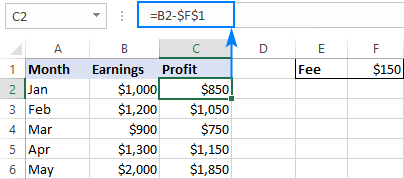If the design of your worksheet does not allow for an extra cell to accommodate the number to be subtracted, nothing prevents you from hardcoding it directly in the formula:

`=B2-150`

## How to subtract percentage in Excel

If you want to simply subtract one percentage from another, the already familiar minus formula will work a treat. For example:

`=100%-30%`

Or, you can enter the percentages in individual cells and subtract those cells:

`=A2-B2`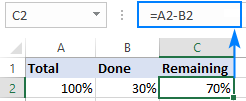If you wish to subtract percentage from a number, i.e. decrease number by percentage, then use this formula:

=Number * (1 - %)

For example, here's how you can reduce the number in A2 by 30%:

`=A2*(1-30%)`

Or you can enter the percentage in an individual cell (say, B2) and refer to that cell by using an absolute reference:

`=A2*(1-\$B\$2)`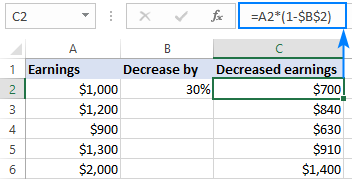## How to subtract dates in Excel

The easiest way to subtract dates in Excel is to enter them in individual cells, and subtract one cell from the other:

=End_date - Start_date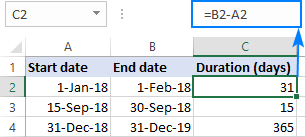You can also supply dates directly in your formula with the help of the DATE or DATEVALUE function. For example:

`=DATE(2018,2,1)-DATE(2018,1,1)`

`=DATEVALUE("2/1/2018")-DATEVALUE("1/1/2018")`

## How to subtract time in Excel

The formula for subtracting time in Excel is built in a similar way:

=End_time-Start_time

For example, to get the difference between the times in A2 and B2, use this formula:

`=A2-B2`

For the result to display correctly, be sure to apply the Time format to the formula cell: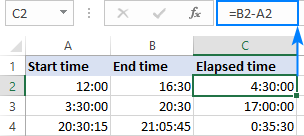You can achieve the same result by supplying the time values directly in the formula. For Excel to understand the times correctly, use the TIMEVALUE function:

`=TIMEVALUE("4:30 PM")-TIMEVALUE("12:00 PM")`

## How to do matrix subtraction in Excel

Suppose you have two sets of values (matrices) and you want to subtract the corresponding elements of the sets like shown in the screenshot below: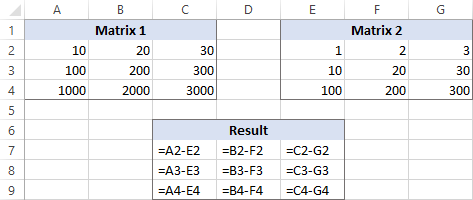Here's how you can do this with a single formula:

1. Select a range of empty cells that has the same number of rows and columns as your matrices.
2. In the selected range or in the formula bar, type the matrix subtraction formula:
`=(A2:C4)-(E2:G4)`
3. Press Ctrl + Shift + Enter to make it an array formula.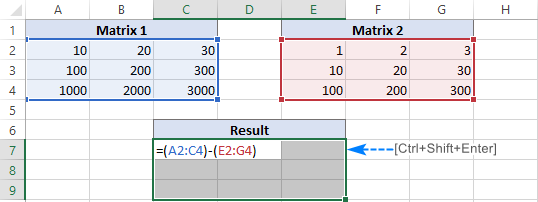The results of the subtraction will appear in the selected range. If you click on any cell in the resulting array and look at the formula bar, you will see that the formula is surrounded by {curly braces}, which is a visual indication of array formulas in Excel: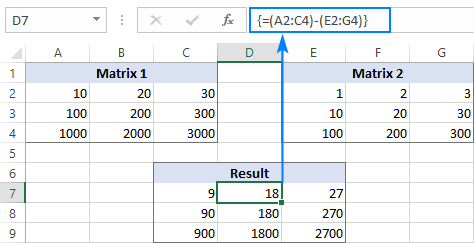If you do not like using array formulas in your worksheets, then you can insert a normal subtraction formula in the top leftmost cell and copy in rightwards and downwards to as many cells as your matrices have rows and columns.

In this example, we could put the below formula in C7 and drag it to the next 2 columns and 2 rows:

`=A2-C4`

Due to the use of relative cell references (without the \$ sign), the formula will adjust based on a relative position of the column and row where it is copied: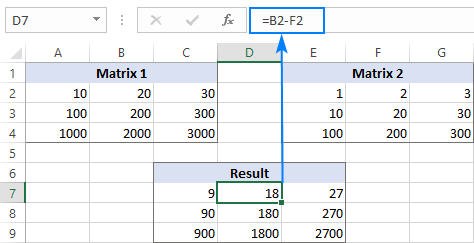## Subtract text of one cell from another cell

Depending on whether you want to treat the uppercase and lowercase characters as the same or different, use one of the following formulas.

### Case-sensitive formula to subtract text

To subtract text of one cell from the text in another cell, use the SUBSTITUTE function to replace the text to be subtracted with an empty string, and then TRIM extra spaces:

TRIM(SUBSTITUTE(full_text, text_to_subtract,""))

With the full text in A2 and substring you want to remove in B2, the formula goes as follows:

`=TRIM(SUBSTITUTE(A2,B2,""))`

As you can see, the formula works beautifully for subtracting a substring from the beginning and from the end of a string: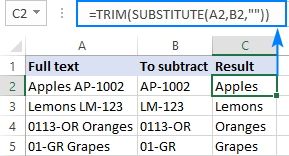If you want to subtract the same text from a range of cells, you can "hard-code" that text in your formula.

As an example, let's remove the word "Apples" from cell A2:

`=TRIM(SUBSTITUTE(A2,"Apples",""))`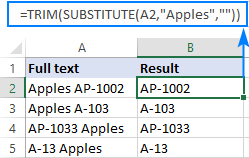For the formula to work, please be sure to type the text exactly, including the character case.

### Case-insensitive formula to subtract text

This formula is based on the same approach - replacing the text to subtract with an empty string. But this time, we will be using the REPLACE function in combination with two other functions that determine where to start and how many characters to replace:

• The SEARCH function returns the position of the first character to subtract within the original string, ignoring text case. This number goes to the start_num argument of the REPLACE function.
• The LEN function finds the length of a substring that should be removed. This number goes to the num_chars argument of REPLACE.

The complete formula looks as follows:

TRIM(REPLACE(full_text, SEARCH(text_to_subtract, full_text), LEN(text_to_subtract),""))

Applied to our sample data set, it takes the following shape:

`=TRIM(REPLACE(A2,SEARCH(B2,A2),LEN(B2),""))`

Where A2 is the original text and B2 is the substring to be removed.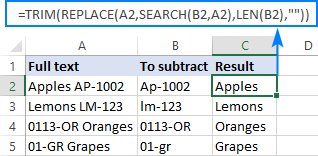## Subtract one list from another

Supposing, you have two lists of text values in different columns, a smaller list being a subset of a larger list. The question is: How do you remove elements of the smaller list from the larger list?

Mathematically, the task boils down to subtracting the smaller list from the larger list:

Larger list: {"A", "B", "C", "D"}

Smaller list: {"A", "C"}

Result: {"B", "D"}

In terms of Excel, we need to compare two lists for unique values, i.e. find the values that appear only in the larger list. For this, use the formula explained in How to compare two columns for differences:

`=IF(COUNTIF(\$B:\$B, \$A2)=0, "Unique", "")`

Where A2 is the first cells of the larger list and B is the column accommodating the smaller list.

As the result, the unique values in the larger list are labeled accordingly: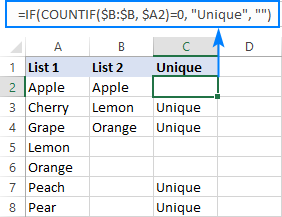And now, you can filter the unique values and copy them wherever you want.

That's how you subtract numbers and cells in Excel. To have a closer look at our examples, please feel free to download our sample workbook below. I thank you for reading and hope to see you on our blog next week!

## Practice workbook

Subtraction formula examples (.xlsx file)

## You may also be interested in

1. I want to subtract 2 filtered rows having 4 coloumns. and i want to keep the subtraction action as is. Whenever i change the filtered criterions, the formula should apply automatically again and sow the result. Something similar to subtotal function, but i want it for subtraction function. how can i do it?

2. Is there a formula where we can continually subtract an amount from a number until the initial number is less than the subtracted number and stop?

Example: 94831 - 10000 but answer will show 4831.

3. I would like to know if there is a way to set up a formula in Excel spreadsheet whereby each week the number in the cell/column reduces by 1 = eg 42 -1 = 41 - 1 =40 and so on every time you open the spreadsheet?

4. Hi, I have a question. If I want to take the number from sheet 2(e.g. B13), and use it in the sheet 1, I start to type

='sheet 2'!B13

in sheet 1,
What if I want the number in sheet 2(from C2 to H7), (in a diagonal way), should I type

='sheet 2'!C2
='sheet 2'!COLUMN(C+1),ROW(2+1)
='sheet 2'!COLUMN(C+2),ROW(2+2)
='sheet 2'!COLUMN(C+3),ROW(2+3)
='sheet 2'!COLUMN(C+4),ROW(2+4)
='sheet 2'!COLUMN(C+5),ROW(2+5)

='sheet 2'!C2
='sheet 2'!D3
='sheet 2'!E4
='sheet 2'!F5
='sheet 2'!G6
='sheet 2'!H7

I know I am wrong, and I want a faster way to deal with the data.😭

EXTRA QUESTION......
What if one of the data from C2 to H7 is 0(zero), how can it automatically skip it and jump to the next one?🤔

5. Hi,
I want a formula to calculate and find the difference between two different rows and columns, For eg, assume cells from rows B5:E5 is 4 and column F1:F4 is 4. now i want to calculate and find the difference between these on F5 and the result should be 0 on F5. Can you suggest a formula?

• Hi!
To calculate the difference between the ranges, I suggest using the SUM function:

=SUM(B5:E5)-SUM(F1:F4)
or
=SUM(B5:E5-F1:F4)

• What formula
Would I use to subtract the quantity of items sold column from the quantity items in stock column. For example: quantity available is 5. Quantity sold is 3. Which formula would I use to make the quantity available column change to 2?

• The available quantity is the difference between the purchased goods and the sold goods.

6. A1 indicates the number of community service hours, if the community service hour goal of 500 hours was met. Create a function that will identify if the goal was achieved